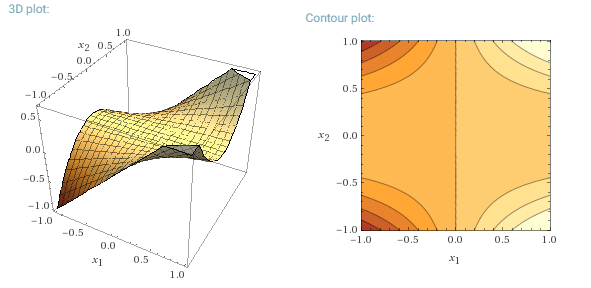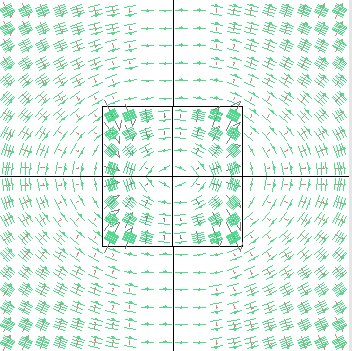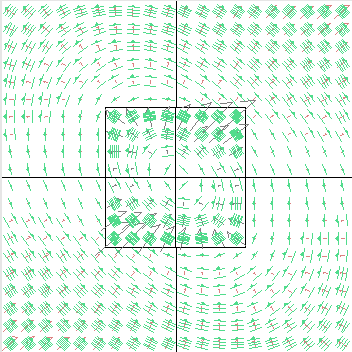References here and here.

The gradient of a multivariate function (or scalar field), $$f(x_1,x_2,\dots,x_n)$$ is denoted by $$\nabla f.$$ If forms a vector field. The gradient points in the direction of the greatest rate of increase of the function, and its magnitude is the slope of the graph in that direction. The components of the gradient in coordinates are the coefficients of the variables in the equation of the tangent space to the graph.

For example, the function

$f(x_1,x_2)=x_1x_2^2\tag 1$Now, the gradient forms a vector field. Dotting the vector field at each point $$x$$ with a vector $$\mathbf v$$ results in the directional derivative.

$\nabla f=\frac{\partial f}{\partial x_1}\mathbf i+\frac{\partial f}{\partial x_2}\mathbf j + \frac{\partial f}{\partial x_3}\mathbf k$

In the case of Eq. 1,

\begin{align} \nabla f&=\frac{\partial f}{\partial x_1}\mathbf i+\frac{\partial f}{\partial x_2}\mathbf j\\[2ex] &=x_2^2 \;\mathbf i + 2x_1x_2\; \mathbf j \tag 2 \end{align}

and it looks like this:shown with arrows (contravariant) and stacks (covariant). It makes a lot of sense: the direction of fastest climb along the first plot goes from the upper left hand-corner to the lower right-hand corner, and from the lower left-hand corner to the upper right-hand corner.

If we were to look for a different coordinate system, say for $$\mathbb R^2,$$ the transformation matrix encapsulating, for example,

\begin{align} \mathbf A &=\begin{bmatrix}T(\mathbf e_1) & T(\mathbf e_2)\end{bmatrix}\\[2ex] &=\begin{bmatrix}2&5\\3&1 \end{bmatrix} \end{align}

implies that $$\mathbf e_1=\begin{bmatrix}1&0\end{bmatrix}^\top$$ will become $$\mathbf \varepsilon_1=\begin{bmatrix}2&3\end{bmatrix}^\top;$$ and likewise, $$\mathbf e_2=\begin{bmatrix}0&1\end{bmatrix}^\top$$ will become $$\mathbf \varepsilon_2=\begin{bmatrix}5&1\end{bmatrix}^\top$$

These new basis vectors are substantially longer than the original standard basis. Therefore, if we were to express in the new basis a vector such as $$\mathbf v=\begin{bmatrix}3&3\end{bmatrix}^\top,$$ which is the sum of $$3\mathbf e_1+3\mathbf e_2,$$ it stands to reason that the coordinates of the vector now are going to be much smaller than $$\begin{bmatrix}3&3\end{bmatrix}^\top.$$ It is as though the longer new basis vectors cover spaces in longer strides. So the transforming matrix will not be the one used above to transform the original basis vectors, but rather its inverse:

$\mathbf A^{-1}=\begin{bmatrix}2&5\\3&1 \end{bmatrix}^{-1}=\frac{-1}{13}\begin{bmatrix}1&-5\\-3&2\end{bmatrix}$

hence

$\frac{-1}{13}\begin{bmatrix}1&-5\\-3&2\end{bmatrix}\begin{bmatrix}3\\3\end{bmatrix}=\begin{bmatrix}0.9\\0.2\end{bmatrix}$

Therefore, the longer the basis vectors, the shorter the expression of individual vectors.

But what would happen to the vector field above (Eq 2)?

We would apply the chain rule to get a new gradient, $$\bar\nabla f:$$

\begin{align} \bar \nabla f &= \left(\frac{\partial f}{\partial x_1}\,\frac{\partial x_1}{\partial \bar x_1}+ \frac{\partial f}{\partial x_2}\,\frac{\partial x_2}{\partial \bar x_1}\right) \mathbf p + \left(\frac{\partial f}{\partial x_1}\,\frac{\partial x_1}{\partial \bar x_2}+ \frac{\partial f}{\partial x_2}\,\frac{\partial x_2}{\partial \bar x_2}\right) \mathbf q \tag 3\\ &=f_{x_i}\frac{\partial x_i}{\partial \bar x_j} \end{align}

where $$\mathbf p$$ and $$\mathbf q$$ are the unit vectors along the new basis $$\{\mathbf \varepsilon_1,\mathbf\varepsilon_2\},$$ and $$\bar x_i$$ the new coordinates. But from the inverse of the transformation matrix

\begin{align} \bar x_1&=\frac{-1}{13}x_1+ \frac{5}{13}x_2\\[2ex] \bar x_2&=\frac{3}{13}x_1+ \frac{-2}{13}x_2\\[2ex] \end{align}

and viceversa

\begin{align} x_1&=2 \bar x_1+ 5 \bar x_2\\[2ex] x_2&=3\bar x_1+ 1 \bar x_2\\[2ex] \end{align}

Hence,

\begin{align} \frac{\partial x_1}{\partial \bar x_1}&=2\\[2ex] \frac{\partial x_2}{\partial \bar x_1}&=3\\[2ex] \frac{\partial x_1}{\partial \bar x_2}&=5\\[2ex] \frac{\partial x_2}{\partial \bar x_2}&=1\\[2ex] \end{align}

Here we see how Eq 3 tells us now that the shorter the basis vectors in relation to the new basis, the steeper the gradient. It makes intuitive sense: a tiny change in the much larger vectors will produce a large change in the function!

Combining Eq 2 with Eq 3,

$\bar \nabla f =\left(2\times x_2^2+ 3\times 2\,x_1x_2\right) \mathbf p + \left(5\times x_2^2+ 1\times 2\,x_1x_2\right) \mathbf q =\left(2x_2^2+ 6x_1x_2\right) \mathbf p + \left(5x_2^2+ 2x_1x_2\right) \mathbf q$a plot similar to the first gradient field, although rotated.